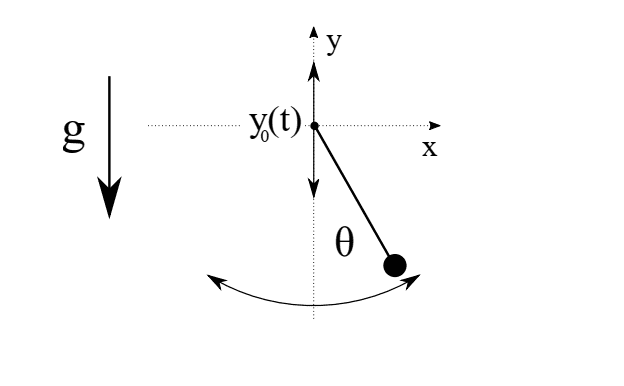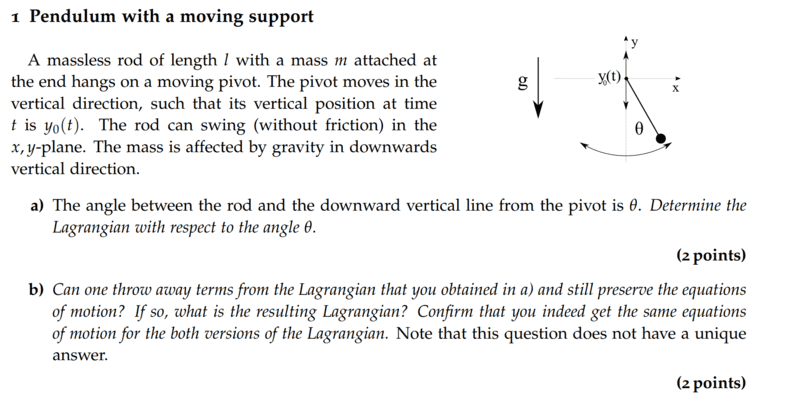# Pendulum with a moving support

Lambda96
Homework Statement:
Can one throw away terms from the Lagrangian that you obtained in a) and still preserve the equations of motion?
Relevant Equations:
##L=\frac{1}{2}m(\dot{\theta}^2l^2-2\dot{\theta}lsin\theta A\omega sin(\omega t)+A^2\omega^2sin^2(\omega t))+mg(\dot{\theta}lsin\theta-A\omega sin(\omega t))##In problem 1 I assumed that the suspension moves up and down in an oscillating manner, so ##y_0(t)=Acos(\omega t)##

I am not quite sure about task 2, but I would say you can remove the motion of the suspension and the motion of the system would not change noticeably, as I assume that the suspension is moving at constant speed and therefore no external force is acting on the system and thus not affecting its motion. So whether the suspension moves or not does not change the typical motion of the pendulum.

Homework Helper
Gold Member
Sorry, I don't get it. What are tasks 1 and 2 and problem 1 that you are referring to?

•BiGyElLoWhAt
Gold Member
Firstly, it's not really clear what you're asking. To get better help, you should provide the problem statement (I believe that's part of the prefilled bits when you post a question, at least it used to be).

If I ignore everything and just answer the question in the homework statement:
It seems like it's asking if you have any terms in the Lagrangian that disappear when you calculate the equations of motion. So when you take your partial derivatives, are there any irrelevant terms. With what you provided, that seems like about as good an answer as what you can expect.

Some clarity might offer better solutions.

Also, if you assumed the support of the pendulum moves Acos(wt), the speed isn't constant. If the base moves with constant frequency, it seems like you're dealing with a driven system.

Lambda96
Sorry, I had unfortunately uploaded the wrong screenshot, here is the correct screenshot with the complete taskHomework Helper
Gold Member
2022 Award
... I assumed that the suspension moves up and down in an oscillating manner, so ##y_0(t)=Acos(\omega t)##
Is that a reasonable assumption?

On the diagram, there are up/down arrows at the pendulum's pivot. But I don't see how you can use that as justification for assuming anything about the specific form of ##y_0(t)##. Or is there some contextual information missing?

Lambda96
You're right, there's nothing about it in the assignment, so unfortunately I can't use a statement like that.

But the suspension itself does not depend on the angle ##\theta##, so I could take the term with the suspension out of the equation without changing the equation of motion.

Gold Member
Take your general Lagrangian, it should have ##y## and ##\dot{y}## terms.
Pick your 2 coordinates you're solving for EOM (there are 2 degrees of freedom, hence 2 EOM).
Calculate the partial derivatives, and note any terms that go to zero when you take the derivatives. Are there any terms that go to zero in both EOM calculations. I suppose maybe some terms could just cancel out as well, if circumstances permit it.
Edit:
Maybe the question is only concerned with the EOM of theta? "Determine the Lagrangian w.r.t. the angle ##\theta##"
However, from where I'm sitting, we have ##L(y,\dot{y},\theta,\dot{\theta})## and EOM for both ##y## and ##\theta##
I suspect this is the case, however. So maybe you're on the right track. You know your professor better than I do, so maybe you can figure out what (s)he is after. The wording is ambiguous to me.

Last edited:
•Lambda96
Homework Helper
Gold Member
2022 Award
Pick your 2 coordinates you're solving for EOM (there are 2 degrees of freedom, hence 2 EOM).
##y_0(t)## is some predefined (supplied) function.

Once ##\theta## is known, the y-coordinate of the pendulum's mass can be geometrically determined from ##\theta, ~y_0(t)## and ##L##.

So surely there is only one degree of freedom (and one EoM).

•Lambda96 and BiGyElLoWhAt
Gold Member
##y_0(t)## is some predefined (supplied) function.
I suppose that's the point of the subscript? I didn't necessarily read it that way. However, I went through the problem 2 ways, one of which effectively having the pivot be in pseudo free fall and just let the position be ##y(t)##, and the EOM are pretty hairy, so that would probably make sense.

•Lambda96
Homework Helper
Gold Member
2022 Award
I suppose that's the point of the subscript?
It's a potentially confusing use of symbols by whoever wrote the original question.

##y_0## would typically be used as the symbol for the initial value of ##y## (i.e. ##y_0## would be a constant), not some variable.

Here, I suspect the 'nought' may be intended to be the letter 'O', to denote the origin of a (moving) coordinate system where the pendulum's pivot is at the origin. ##y_p## might have been a better choice!

•Lambda96 and BiGyElLoWhAt
Homework Helper
Gold Member
2022 Award
@Lambda96. I’m guessing what you have to do might be this:

For part a), express the Lagrangian in terms of ##y_0, ~\theta##, their derivatives, and constants.

To make sense of part b) you could state that you will consider the special case where ##\ddot {y_0}=0##, i.e. the pivot is not accelerating. Some terms in the Lagrangian should vanish. (The EoM - which is not actually required - would then be the same as for a standard simple pendulum.)

•Lambda96
Gold Member
Also worth noting, if you assume small angle from the get go, your horizontal component of the velocity becomes ##\ell\dot{\theta}##. This gives the same result as what @Steve4Physics provided.

•Lambda96
Homework Helper
Gold Member
2022 Award
Also worth noting, if you assume small angle from the get go, your horizontal component of the velocity becomes ##\ell\dot{\theta}##. This gives the same result as what @Steve4Physics provided.
I didn't provide a result!

My suggestion for how to interpret part b), was to consider the case where ##\ddot {y_0}=0##. This does not relate to the small angle approximation; a pendulum with a non-accelerating pivot could still have a large angular amplitude.

Of course, if the EoM is required. it would make sense to use the small angle approximation (if appropriate) in order to avoid dealing with elliptic functions.

Lambda96
Thank you BiGyElLoWhAt and Steve4Physics for your help 👍

I have now done task 1 again with ##y_0(t)##

For ##x## and ##y## I assumed the following

$$x=lsin(\theta) ,\qquad \dot{x}=\dot{\theta}lcos(\theta),\qquad \dot{x}^2=\dot{\theta}^2l^2cos^2(\theta)$$

$$y=-lcos(\theta)+y_0(t) ,\qquad \dot{y}=\dot{\theta}lsin(\theta)+\dot{y_0}(t),\qquad \dot{y}^2=\dot{\theta}^2l^2sin^2(\theta)+2\dot{\theta}lsin(\theta)\dot{y_0}(t)+\dot{y_0}^2(t),\qquad$$

I then obtained the following as a Lagrangian.

$$L=\frac{1}{2}m\Bigl(\dot{\theta}^2l^2+2\dot{\theta}lsin(\theta)\dot{y_0}(t)\Bigr)+mg\Bigl(lcos(\theta)-y_0(t)\Bigr)$$

For task b I then wanted to form the Euler-Lagrange equation ##\frac{\partial L}{\partial \theta}-\frac{d}{dt}\frac{\partial L}{\partial \dot{\theta}}=0## and got the following.

$$\frac{\partial L}{\partial \theta}-\frac{d}{dt}\frac{\partial L}{\partial \dot{\theta}}=m\dot{\theta}lcos(\theta)\dot{y_0}(t)- mglsin(\theta)-m\ddot{\theta}l^2-mlcos(\theta)\dot{y_0}(t)-mlsin(\theta)\ddot{y_0}(t)$$

Unfortunately, only now the terms ##m\dot{\theta}cos(\theta)\dot{y_0}(2)## and ##mlcos(\theta)\dot{y_0}(t)## cause me problems, as they would not disappear if I assume ##\ddot{y_0}(t)=0##.

Last edited:
Homework Helper
Gold Member
$$y=-lcos(\theta)+y_0(t) ,\qquad \dot{y}=\dot{\theta}lsin(\theta)+\dot{y}(t),\qquad \ddot{y}=\dot{\theta}^2l^2sin^2(\theta)+2\dot{\theta}lsin(\theta)\dot{y}(t)+\dot{y}^2(t),\qquad$$
How do you take derivatives with respect to time?
If ##~ \dot{y}=\dot{\theta}l\sin(\theta)+\dot{y}(t),##
then ##~~\ddot{y}=\ddot{\theta}l\sin(\theta)+\dot \theta^2l\cos(\theta)+\ddot{y}(t),~##no?

Also, your notation is confusing. What is the difference between ##y## and ##y(t)##? Isn't the first ##y## also a function of time?

Last edited:
•Steve4Physics
Homework Helper
Gold Member
2022 Award
I have now done task 1 again with ##f_0(t)##
Is "##f_0##" a typo'?

And in addition to what @kuruman just said:
##x=lsin(\theta)##. OK
##\dot{x}=\dot{x}lcos(\theta)##. Is that a typo’?
##\ddot{x}=\dot{\theta}^2l^2cos^2(\theta)##. Are you sure about that? (product rule)

You also changed from using ##y_0## to just ##y##, which could be a source of confusion (e.g. if ##y## is the y-coordinate of the pendulum's mass).

•kuruman
Homework Helper
Gold Member
##\dot{x}=\dot{x}lcos(\theta)##. Is that a typo’?
In fact, if that is true, it follows that ##\cos\theta=1/l## which is not only dimensionally incorrect, but also means that the pendulum is fixed at a constant angle and does not swing at all. Truth be told, I didn't look at the ##x## equations too closely when I posted #15.

Lambda96
Sorry, I made some typos in post #14 unfortunately

##f_0(t)## should of course be ##y_0(t)## and ##\ddot{x}## and ##\ddot{y}## should actually be ##\dot{x}^2## and ##\dot{y}^2## I have now changed the details in post 14.

Homework Helper
Gold Member
Unfortunately, only now the terms ##m\dot{\theta}cos(\theta)\dot{y_0}(2)## and ##mlcos(\theta)\dot{y_0}(t)## cause me problems, as they would not disappear if I assume ##\ddot{y_0}(t)=0##.
Your notation still leaves something to be desired. What on Earth is ##\dot{y_0}(2)##? To me it says "##\dot{y_0}(t)##" evaluated at ##t = 2## in whatever units.

Homework Helper
Gold Member
2022 Award
I have now changed the details in post 14.
Are you sure your current Post #14 Lagrangian:
##L=\frac{1}{2}[m\Bigl(\dot{\theta}^2l^2+2\dot{\theta}lsin(\theta)\dot{y_0}(t)\Bigr)+mg\Bigl(lcos(\theta)-y_0(t)\Bigr)##
is correct?

We know ##\dot y= \dot{\theta}lsin(\theta)+\dot{y_0}(t)##. Part of the kinetic energy expression must contain ##\dot{y_0}(t)^2##.

On a more general point, I think the original (part b)) question is poorly written. My opinion (but note I’m often wrong!) is that part b) should say:

“Are there any situations in which we can throw away terms from the Lagrangian..."​

Taking ##\ddot {y_0(t)} = 0## is such a situation.

(A different, interesting, but probably not relevant, situation occurs when ##\ddot {y_0(t)} = \text {constant}## . In that case, the equation of motion should reduce that of simple (fixed pivot) pendulum but in a gravitational field of strength ##g+\ddot {y_0(t)}##.)

Gold Member
I didn't provide a result!
You didn't explicitly provide a result, but in the special case ##\ddot{y}_0(t) = 0##, it clearly simplifies to the ordinary pendulum, and the ##\theta## EoM are then those of the standard pendulum. In this case, using general ##y(t)## and taking small angle right off the bat, so ##\dot{x} = \ell \dot{\theta}## also yields the same standard equations for theta.

Last edited:
Homework Helper
Gold Member
2022 Award
You didn't explicitly provide a result, but in the special case y = 0, it clearly simplifies to the ordinary pendulum, and the ##\theta## EoM are then those of the standard pendulum.
Agreed - but note that you mean ##\ddot y_0=0##, since ##y## (unsubscripted) would typically be interpreted as the y-coordinate of the mass, rather than that of the pivot.

•BiGyElLoWhAt
Gold Member
2022 Award
Homework Statement:: Can one throw away terms from the Lagrangian that you obtained in a) and still preserve the equations of motion?
Relevant Equations:: Result from task 1 ##L=\frac{1}{2}m(\dot{\theta}^2l^2-2\dot{\theta}lsin\theta A\omega sin(\omega t)+A^2\omega^2sin^2(\omega t))+mg(\dot{\theta}lsin\theta-A\omega sin(\omega t))##

View attachment 318122

In problem 1 I assumed that the suspension moves up and down in an oscillating manner, so ##y_0(t)=Acos(\omega t)##

I am not quite sure about task 2, but I would say you can remove the motion of the suspension and the motion of the system would not change noticeably, as I assume that the suspension is moving at constant speed and therefore no external force is acting on the system and thus not affecting its motion. So whether the suspension moves or not does not change the typical motion of the pendulum.
I've not followed the discussion in detail, but shouldn't the Lagrangian be
$$L=\frac{m}{2} (l^2 \dot{\theta}^2-2 l \dot{y}_0 \dot{\theta} \sin \theta + \dot{y}_0^2) + m g (y_0+l \cos \theta)?$$
Then you can omit everything of the form ##\frac{\mathrm{d}}{\mathrm{d} t} \Omega(t,\theta)##. Here I only see the terms which don't depend on ##\theta## and ##\dot{\theta}## at all, i.e., an equivalent Lagrangian is
$$L=\frac{m}{2} (l^2 \dot{\theta}^2-2 l \dot{y}_0 \dot{\theta} \sin \theta) + m g l \cos \theta.$$
$$\ddot{\theta}=\frac{\ddot{y}_0-g}{l} \sin \theta.$$